×
Get Full Access to UA - CH 102 - Study Guide - Midterm
Get Full Access to UA - CH 102 - Study Guide - Midterm

×

UA / Chemistry / CH 102 / What accounts for he difference between density and volume?

# What accounts for he difference between density and volume? Description

Test 1 Study Guide Chapters 12-13

## What accounts for he difference between density and volume?CHAPTER 12 LIQUIDS, SOLIDS, AND INTERMOLECULAR FORCES 12.1 Structure determines property

∙ The structure of a molecule determines the strength of its intermolecular  forces (attractive forces between all molecules and atoms)

∙ State of matter (gas, liquid, or solid) depends on the magnitude of  intermolecular forces between the particles relative to the amount of thermal  energy in the sample

o Matter with high thermal energy relative to intermolecular forces tend  to be gaseous

o Matter with low thermal energy relative to intermolecular forces tend  to be liquid or solid

12.2 Solids, liquids, and gas: A Molecular Comparison

Phase

Temp. (C)

Density

(g/mol)

Molar volume

Molecular view

Gas (steam)

100

5.9 x 10-4

30.5 L

Liquid (water)

20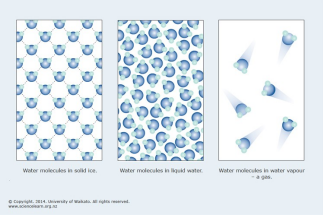0.998

18 mL

Solid (ice)

0

0.917

19.6 mL

## What causes dispersion forces?We also discuss several other topics like What is the correlation between common sense and hindsight?

∙ Differences in density and volume are due to the space between the  molecules, which increases with temperature

∙ Thermal energy can overcome the intermolecular forces to allow movement  of molecules

o Thus liquids and gases can take the shape of their containers ∙ You can change between states by changing the temperature and/or pressure o Increases in pressure favor denser states (more pressure = gas  liquid)

12.3 Intermolecular Forces

∙ The result of charges, partial charges, and temporary charges interacting at  large distances If you want to learn more check out What is agrarian america?

∙ Greater distance = weaker force (this is why bonding forces are stronger –  they occur within the molecule, not between molecules)

## What refers to the resistance of liquid to flow?∙ Dispersion forces result from changes in electron distribution within  molecules and atoms

o Present in all molecules and atoms We also discuss several other topics like ­ what are politics?

o At any instant, one side may have a negative charge and the other  may have a positive charge. This charge separation is called temporary dipole

o The temporary dipole of one atom causes temporary dipoles on nearby atoms.

o The positive end of one atom is attracted to the negative end on the  other atom resulting in a dispersion force between the atoms

o Magnitude of dispersion depends on how easily the electrons move  within the molecule, which depends on the volume of the electron  cloud We also discuss several other topics like What were computers first use?

Higher molar mass = more electrons over a great volume = greater  dispersion force

o Boiling point increases with strength of intermolecular forces because  more heat is needed to break them apart

o Boiling point increase with surface area exposure because greater  surface area means greater interaction between molecules and thus  greater intermolecular forces

∙ Dipole Dipole Force exist in all polar molecules

o The positive end of one permanent dipole attracts the negative end of  another permanent dipole

o Polar molecules have higher melting and boiling points We also discuss several other topics like What are the 10 input devices?

∙ Hydrogen bonds are intermolecular forces between polar molecules that  contain hydrogen and at least one of the following: fluorine, oxygen, or  nitrogen

o The large electronegative difference between H and F, O, and N cause  the hydrogen atom to have a large partial positive charge within the  atom and the other atom to have a large partial negative charge

o NOTE! The hydrogen bond is not within the molecule, it is between the  H, F, O, and N of different molecules

o Hydrogen bond raise the boiling and melting points even more 12.4 Intermolecular Forces in Action

∙ Surface Tension is the tendency of liquids to minimize their surface area o A molecule at the surface has less molecules with which to interact and is thereby less stable (higher potential energy)

o Increasing surface area requires energy because molecules on the  inside must move towards the outside, reducing their stability We also discuss several other topics like In what way do illnesses determine our lives?

o Surface tension decreases as intermolecular forces decrease o Surface tension is why a paper clips floats and water droplets force  spheres

∙ Viscosity is the resistance of liquid to flow

o Increases with stronger intermolecular forces because molecules that  are more attracted to their neighbors will not flow as easily

o Increases with larger molecular shape because longer molecules  interact over a greater area and become entangled

o Viscosity decreases higher temperatures because thermal energy  partially overcomes intermolecular forces, allowing molecules to flow  more easily

∙ Capillary Action is the ability of a liquid to flow up against gravity up a narrow tube

o Results from:

1) cohesive forces – attraction between molecules in a liquid which  cause the liquid to stay together

2) adhesive forces – attraction between molecules and the surface of  the tube, which cause the liquid to spread out over the tube

a. if adhesive forces > cohesive forces, then the attraction to

the surface draws liquid up the tube and the cohesive force

pulls along the molecules not in direct contact with the walls

b. if cohesive forces > adhesive forces, then the liquid will not  rise

12.5 Vaporization and Vapor Pressure

∙ Process of Vaporization = liquid to gas when molecules gain enough energy  to break free from the surface

o Rate of vaporization increases with:

 increasing temperature – molecules have more energy to break  bonds

 increasing surface area – more molecules at the surface, where  vaporization is higher because they are held less tightly

 decreasing strength of intermolecular forces – molecules are  held less tightly

o volatile liquids vaporize easily

∙ Energetics of Vaporization

o Vaporization is an endothermic process because it requires energy to  overcome the molecular forces

 Condensation is the opposite of vaporization (gas to liquid) and  is exothermic

o ∆HVap = amount of heat required to vaporize 1 mole of a liquid (always  positive)

o The heat of vaporization can be used to calculate the amount of  energy required to vaporize a given mass of a liquid

PRACTICE PROBLEM 1: Calculate the mass of water that can be vaporized at boiling  point with 155kJ of heat

1 mol of water = 18.02 g

Heat of vaporization of water = 40.7 kJ/mol

Heat = 155 kJ

155 kJ x1 mol

40.7 kJ /molx18.02 g

1mol=68.6 g of water

∙ Dynamic Equilibrium is reached when rate of condensation and rate of  vaporization are equal

o In an open container, the water will convert to gas and float away; but  in a sealed container, the gas molecules remain in the container and  eventually convert back to liquid molecules

o Vapor pressure is the pressure of a gas in dynamic equilibrium with its  liquid

 Depends on intermolecular forces (weak forces = high vapor  pressure because the energy can easily overcome the forces)  and temperature

o When a system in dynamic equilibrium is disturbed, it responds in such a way to minimize the disturbance and return to equilibrium

 I.e. if pressure decrease, liquid evaporates to restore pressure ∙ Temperature Dependence of Vapor Pressure & Boiling Point o Boiling point is the temperature at which vapor pressure equals  external pressure

 When a liquid reaches boiling point, thermal energy is enough  for molecules in the interior to convert to gas molecules

o Normal boiling point is the temperature at which vapor pressure equals 1 atm

 100 C for water; but water can boil at a lower temperature if  pressure is lower

 Additional heating will not raise temperature, only increase  boiling rate, until all the liquid has been converted to gas

o When temperature increases, vapor pressure increases because high  thermal energy vaporizes molecules

 Relationship is exponential; given by

P1

Clausius- Clapeyron Equation: ln (

−∆ Hvap

R (1T 1−1T 2 )

P 2) =

∙ gives the relationship between the natural log of vapor

pressure and the inverse of temperature

∙ the slope of the line between the natural log of vapor

pressure and the inverse of temperature determines the

heat of vaporization

∙ Temperatures must be absolute (in Kelvin, so add 273)

PRACTICE PROBLEM 2: The vapor pressure of liquid chloroform is 53.1 kPa at 24.1 C  and 13.3 kPa at -6.30 C. What is the heat of vaporization of chloroform?

Celsius to kelvin  24.1 C = 297.3 K -6.30 C = 266.9 K

ln (

P1

P 2) =

R (1T 1−1T 2 )  ln (53.1 kPa

−∆ Hvap

13.3 kPa ) =

−∆ Hvap

R (1

297.3−1

266.9 )

1.38 (kPa canceled out) =

−∆ Hvap

R(−0.000383 k )

−∆ Hvap

RR = 8.314

J

molx K

-3613 k =

30,043

J

mol or 30.0

kJ

mol

∙ The Critical Point is the temperature and pressure at which gas and liquid  coexist to form a supercritical fluid

o Heating a sealed container cause temperature and pressure to rise  (more molecules become gaseous). This results in the density of gas in the container to increase, but the density of liquid to decrease (as  liquid molecules become gaseous). When the meniscus line between  the two states is gone, they comingle

o Supercritical fluids have gas and liquid properties, and are good  solvents

12.6 Sublimation and Fusion

∙ Sublimation = solid to gas transformation (molecules with high enough  thermal energy break free of the surface and go straight to gas state o Opposite is deposition. Molecules collide with the surface and are  captured by intermolecular forces, going from gas to solid.

∙ Fusion = solid to liquid transformation (aka melting). Increasing temperature  causes molecules to vibrate faster and overcome the intermolecular forces  that hold them in place

o Opposite is freezing.

∙ Heat of Fusion (∆Hfus) is the amount of heat required to melt 1 mol of a solid o Positive because melting is endothermic (requires absorbing heat)  Change in enthalpy for freezing has the same magnitude as  melting but opposite sign because the process is exothermic

∆H = - ∆Hfus

o Heat of fusion is less than heat of vaporization because it takes less  energy since the intermolecular forces are not being completely

separated (like they are during vaporization)

12.7 Heating Curve of Water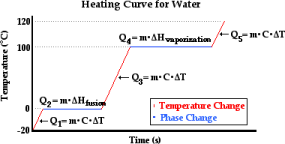∙ Q1

o Ice is warmed (temperature increases) but there is no phase change o Amount of heat required to heat the ice is mC∆T, where m is mass, C is specific heat capacity of ice, and delta T is change in temperature

Ex. How much heat is required to raise 18 g of water from – 25 C to 0  C?

J

g x C )( 0 C - (-25 C))  941 J

∙ Q2

q= mC∆T  (18 g) (2.09

o Adding heat does not change the temperature of the ice and liquid  mixture because added heat is used to transition from solid to liquid o Amount of heat required to convert ice to liquid water is given by q=  n∆Hfus where n is the number of moles and ∆Hfus is the heat of fusion o Heat of fusion for water is 6.02 kJ/mol

∙ Q3

o Here, temperature of the liquid increases and there is no change of  state.

o Amount of heat required to heat the liquid is mC∆T, where m is mass, C is specific heat capacity of liquid, and delta T is change in temperature Ex. How much heat is required to raise 18 g of water from 0 C to 100  C?

J

g x C )( 100 C - 0 C)  7.52 x 103 J

∙ Q4

q= mC∆T  (18 g) (4.18

o Adding heat does not change the temperature of the liquid and gas  mixture because added heat is used to transition from liquid to gas o Amount of heat required to convert liquid water to gas is given by q=  n∆Hvap where n is the number of moles and ∆Hvap is the heat of  vaporization

o Heat of vaporization for water is 40.7 kJ/mol

∙ Q5

o Gas is warmed and increasing temperature does not change state of  matter

o Amount of heat required to heat the steam is mC∆T, where m is mass,  C is specific heat capacity of steam, and delta T is change in  temperature

Ex. How much heat is required to raise 18 g of water from 100 C to 125 C?

J

q= mC∆T  (18 g) (2.01

g x C )( 125 C - 100 C)  904 J

12.8 Water: An Extraordinary Substance

∙ Has a high boiling point (despite low molar mass) because of its molecular  structure

o O – H bonds allow it to form strong hydrogen bonds with other  molecules, giving it a high boiling point

∙ It is bent and highly polar so it has a strong dipole moment o High polarity allows it to dissolve many other polar and ionic  substances by inducing a dipole moment in their molecules

∙ High specific heat capacity allows it to regulate climate temperatures ∙ Expands when it freezes (other substances contract)

o Ice is less dense than water, which is why it floats

CHAPTER 13 PHASE DIAGRAMS AND CRYSTALLINE STRUCTURES  13.2 Phase Diagrams

∙ A phase diagram is a map of the state or phase of a substance as a function  of pressure (on the y axis) and temperature (on the x axis). Made up of: o Regions – conditions where that particular state is stable (solid, liquid,  or gas)

o Lines – representing a set up temperatures and pressures at which a  substance is in equilibrium between two phases

o The Triple Point – the unique set of conditions where all three states of  the substance are stable and in equilibrium

o The Critical Point – the temperature and pressure above which the  substance exists as a supercritical fluid

∙ Changes in temperature and/or pressure is movement within the diagram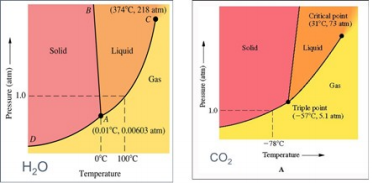13.3 Crystalline Solids

∙ X Ray Diffraction is a lab technique used to look at the arrangement of atoms  and the distance between them

o Crystalline solids are composed of atoms or molecules arranged in  structures with long range orders, with spaces between the atoms.  Light of similar wavelengths form diffraction patterns when it interferes with the atoms

o Braggs Law – for a given wavelength of light, we can measure the  angle that produces constructive interference and calculate the  difference between atomic layers

n = 2a n  number of wavelengths 2a difference  between the two paths

a

sin(θ)=

da  distance d separation between atomic layers nλ

n = 2dsin(θ) or d=

2sinθBraggs Law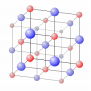13.4 Crystalline Solids: Unit Cells and Basic Structures

∙ Arranged in a crystalline lattice to minimize the energy of particles

o Lattice structure is created when the unit cell (collection of atoms, ions, and molecules) repeats over and over again

o Each atom in a unit cell is identical to the other atoms in the structure o “Cubic Unit Cells” have equal edge lengths and 90 degree angles at

the corners. 3 kinds:

Cubic Cell Name

Atoms

per cell

Crystal

lattice

Crystal

structure

Coordinati on

Number

(# of

atoms

each

atom is in direct

contact

with)

Edge

length

(L in terms of r; radius of the

atom)

Packing

efficiency

(% volume of the structure occupied by the

spheres/ato

ms)

Simple

Cubic

1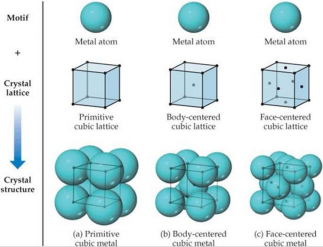6

L = 2r

52%

Body

centered

cubic

2

8

L=4r

√3

68%

Face

centered

cubic

4

12

L= 2r

√2

74%

∙ Edge length can be used to calculate density and volume

PRACTICE PROBLEM 1: Palladium crystallizes in a face-centered cubic unit cell. Its  density is 12.023 g/cm3. Calculate the atomic radius of palladium.

Steps:

1. Calculate the average mass of one atom of Pd:

106.42 mol¯1 ÷ 6.022 x 1023 atoms mol¯1 = 1.767187 x

10¯22 g/atom

2. Calculate the mass of the 4 palladium atoms in the face-centered cubic unit cell:

1.767187 x 10¯22 g/atom times 4 atoms/unit cell = 7.068748 x

10¯22 g/unit cell

3. Use density to get the volume of the unit cell:

7.068748 x 10¯22 g ÷ 12.023 g/cm3 = 5.8793545 x 10¯23 cm3

4. Determine the edge length of the unit cell:

3√5.8793545 x 10ˉ 23cm 3 = 3.88845 x 10¯8 cm

Remember that a face-centered unit cell has an atom in the middle of  each face of the cube. The square represents one face of a face centered cube: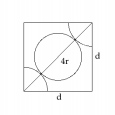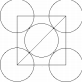Using the Pythagorean Theorem, we find:

d2 + d2 = (4r)2

2d2 = 16r2

r2 = d2 ÷ 8

r = d ÷ √8 or r = d ÷ 2(√2)

r = 1.3748 x 10¯8 cm

PRACTICE PROBLEM 2: Krypton crystallizes with a face-centered cubic unit cell of  edge 559 pm.

a) What is the density of solid krypton?

b) What is the atomic radius of krypton?

c) What is the volume of one krypton atom?

d) What percentage of the unit cell is empty space if each

atom is treated as a hard sphere?

a) Steps:

1) Convert pm to cm:

559 pm x (1 cm/10-10 pm) = 559 x 10¯10 cm = 5.59 x 10¯8 cm

2) Calculate the volume of the unit cell:

(5.59 x 10¯8 cm)3 = 1.7468 x 10¯22 cm3

3) Calculate the average mass of one atom of Kr:

83.798 g/mol divided by 6.022 x 1023 atoms/mol = 1.39153 x

10¯22 g

4) Calculate the mass of the 4 krypton atoms in the face-centered  cubic unit cell:

1.39153 x 10¯22 g times 4 = 5.566 x 10¯22 g

5) Calculate the density (value from step 4 divided by value from step  2):

5.566 x 10¯22 g / 1.7468 x 10¯22 cm3 = 3.19 g/cm3

b) Steps:

r = d ÷ 2√2

r = 5.59 x 10¯8 cm ÷ 2√2

r = 1.98 x 10¯8 cm

c) Steps

V = (4/3) π r3

V = (4/3) (3.14159) (1.98 x 10¯8 cm)3

V = 3.23 x 10¯23 cm3

d) Steps:

1) Calculate the volume of the 4 atoms in the unit cell:

3.23 x 10¯23 cm3 x 4 = 1.29 x 10¯22 cm3

2) Calculate volume of cell not filled with Kr:

1.7468 x 10¯22 cm3 - 1.29 x 10¯22 cm3 = 4.568 x 10¯23 cm3 3) Calculate % empty space:

4.568 x 10¯23 cm3 ÷ 1.7468 x 10¯22 cm3 = 0.2615 x 100% = 26%

o Close packed structures

 Think of crystal structures as layers upon layers of atoms   Simple unit cubic structures are not efficient at packing because  they have square packing, which leaves a lot of room between  atoms:

 Hexagonal Closest Packing is one way to increase efficiency:  align neighboring rows of atoms slightly off center from each  other and put them directly on top of each other. Pattern is ABAB

 Closest Cubic Packing/ Face Centered Packing also increases  efficiency and has pattern ABCABC. Every fourth layer is aligned  with the first.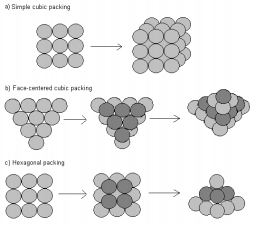13.5 Crystalline Solids: The Fundamental Types

∙ Molecular Crystalline Solids (i.e. ice)

o composed of molecules

o held together by intermolecular forces

o tend to have low to moderately low melting points

∙ Ionic Solids (i.e. table salt, NaCl)

o Composed of ions

o Held together by strong coulombic forces (ionic bonds)

o Much higher melting points because coulombic forces are stronger  than intermolecular

∙ Atomic Crystalline Solids

o Composed of individual atoms

o 3 subcategories:

 Nonbonding atomic solids (i.e. solid Argon)

∙ Held together by weak dispersion forces

∙ Form closest packing structures to maximize interactions

and minimize distance

∙ Very low melting points

∙ Noble gases in their solid form are the only nonbonding

atomic solids

 Metallic atomic solids (i.e. Solid iron)

∙ Held together by metallic bonds (interaction of metal

cations with the sea of electrons that surrounds them) of

different strengths

∙ Tend to form closest packed crystalline structures

∙ Various melting points

 Network covalent atomic solids (i.e. Diamond or graphite)

∙ Held together by covalent bonds

∙ Structures are restricted by geometrical constraints of the

bonds

∙ Do not form closest packed crystalline structures

∙ Very high melting points

13.6 Structure of Ionic Solids

∙ Similar structures to that of unit cells designed to:

o minimize potential energy

o accommodating both charges so the solid is neutral

o accommodates the sizes of the cations and anions

∙ Cations and anions in the solid attract each other

∙ Coordination number is determined by the number of close anion-cation  interactions

o The more similar the radii of the cation and anion, the higher the  coordination number

∙ If the cation: anion ratio is not 1:1, the structure must accommodate this o Many with a cation: anion ratio of 1:2 take on the fluorite structure

Cations occupy the lattice sites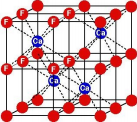of face centered cubic

structures and anions occupy

the tetrahedral holes directly

beneath each corner atom

o Many with a cation: anion ratio of 2:1 take on the antifluorite structure

Anions occupy the lattice sites of face centered cubic structures  and cations occupy the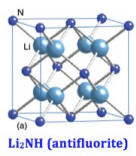tetrahedral holes directly

beneath each corner atom

13.7 Network Covalent Atomic Solids: Carbon  and Silicates

∙      Carbon exists in several forms o Graphite consists of flat sheets of carbon  atoms covalently bonded together as

interconnected hexagonal rings

 Bond length between atoms within a sheet is 142 pm

 Separation between sheets is 341 pm; no covalent bonds, only  dispersion forces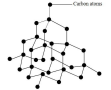 Electrons in the sheets make it a good conductor of electricity  along the sheets

 Putting lots of pressure turns graphite into diamond

o Diamonds structure consists of carbon atoms covalently bonded to four other carbon atoms at the corners of a tetrahedron

 High melting point

 electrons are not free to flow, so it is not a good conductor of  electricity

o Fullerenes are soccer ball shaped clusters of carbon atoms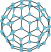 Solid at room temperature

 Held together by dispersion forces

 Soluble in nonpolar solvents, and form solutions of different  colors

o Nanotubes are sheets of interconnected C6 rings that take the shape of a cylinder with closed ends that can be opened when heated

 Single wall nanotubes (SWNT) have one layer of interconnected  carbon rings forming the walls

 Multiwall nanotubes (MWNT) have concentric layers of carbon  forming the walls

o Silicates are extended arrays of silicon and carbon

 Most common network of covalent atomic solids

 Silicon atoms bond to four oxygen atoms with a single sigma  covalent bond, forming a tetrahedron. Each oxygen forms a  second covalent bond to a different Silicon atom, forming a  three dimensional quartz (SiO2 – silica)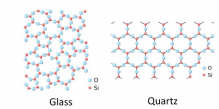Page ExpiredIt looks like your free minutes have expired! Lucky for you we have all the content you need, just sign up here